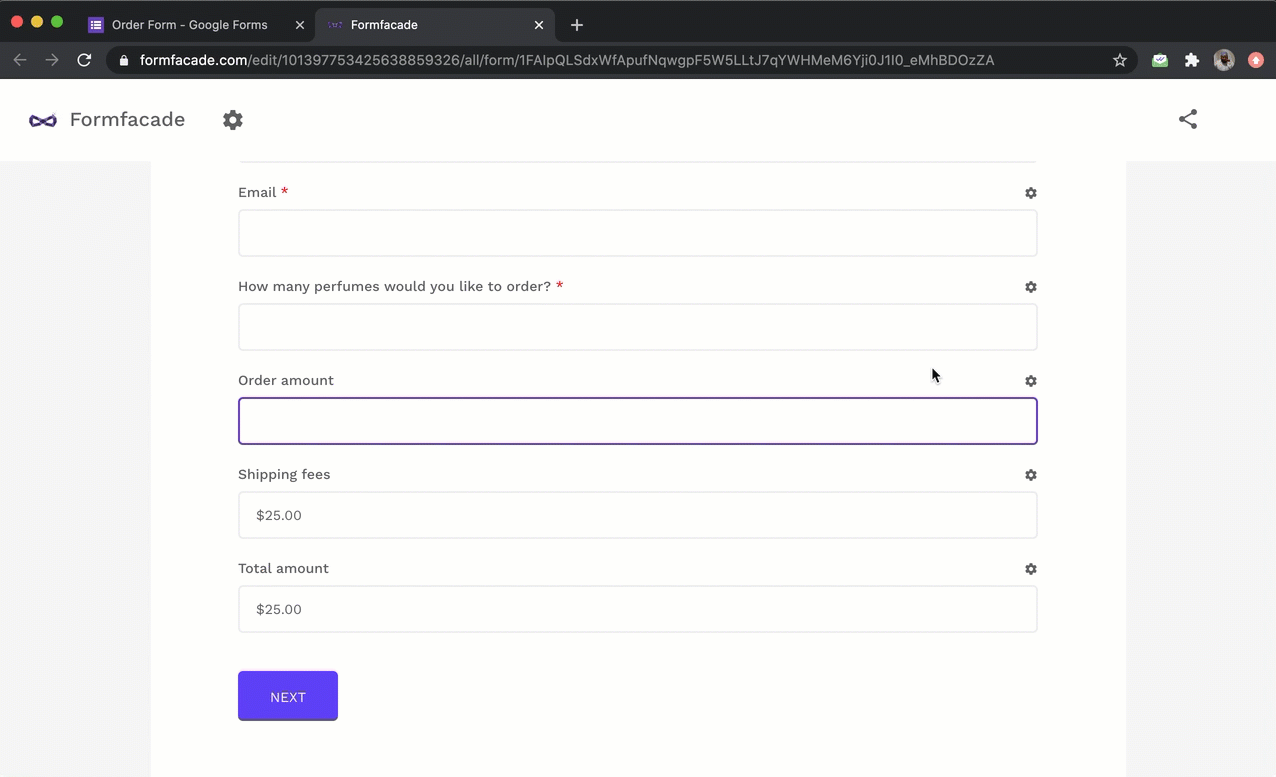### Google Forms does not support dynamic calculations, but you can use Formfacade's calculate field option to automatically compute and display the data in real-time to the user filling the form.

Calculated fields allow you to compute a field with values from other fields. Calculated fields can perform arithmetic on number fields, manipulate text & date. For example, you can calculate the total amount in order form based on the order quantity and shipping option chosen by the user.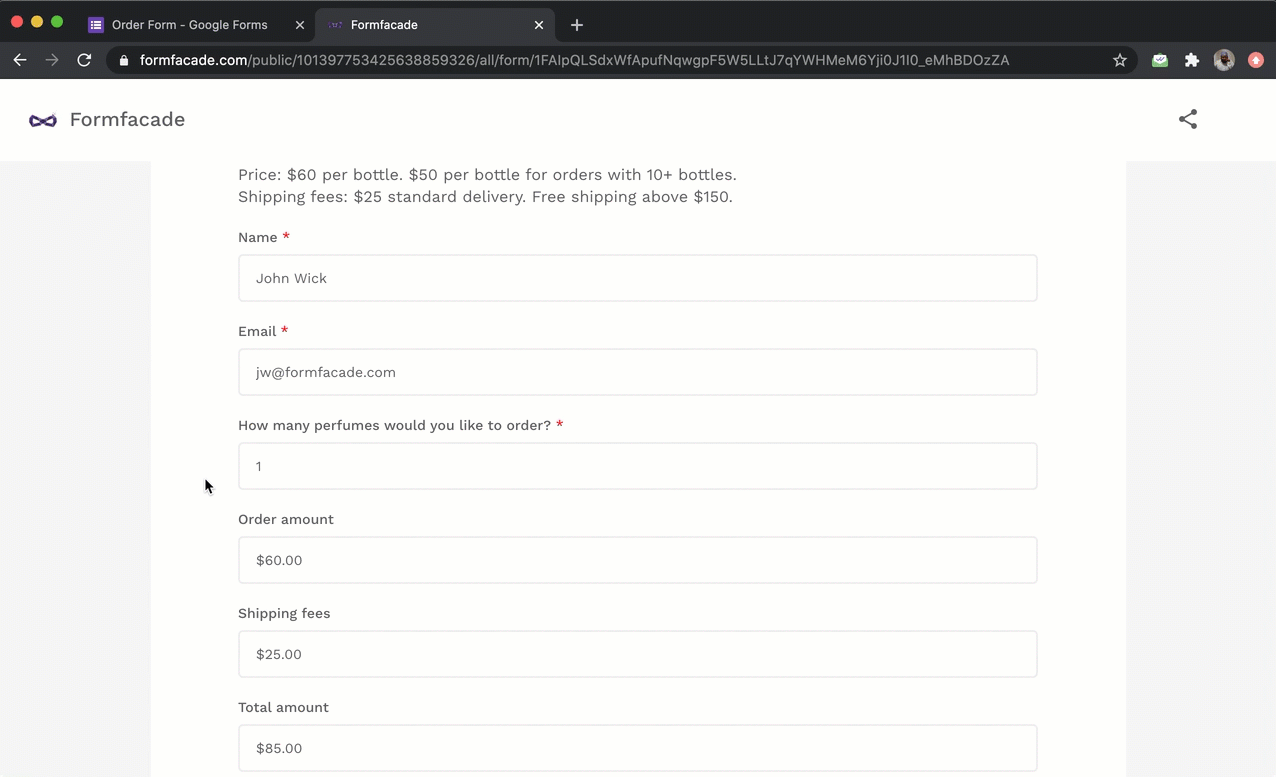#### Important

We have simplified the calculations for standard use cases such as calculating total amount and displaying order summary in order forms, calculating scores and providing verbal assessments for surveys and questionnaires.

Order forms

Let's create a simple order form and learn how to add a calculated field to compute and display the order amount to the user filling the form. Users can order one or more bottles of perfume using the order form. The standard price is \$60, but if they order 10 or more bottles of the perfume, the price per bottle is \$50. There is a standard shipping fees of \$25, but this fees is waived off for orders above \$150.

Calculations to be added in the order form:
Product price = \$60 for < 10 bottles of perfume; \$50 for >=10 bottles of perfume
Order amount = Quantity * Price
Shipping fees = \$0 for order amount > 150; \$25 for order amount =< 150
Total amount = Order amount + Shipping fees

Google Forms does not support dynamic calculations, but you can use Formfacade's calculate field option to automatically compute and display the data in real-time to the user filling the form.

Open your Google Forms > Click on the addon icon > Select Formfacade > Select Customize this form > Click on the Proceed button to open the Formfacade customize interface.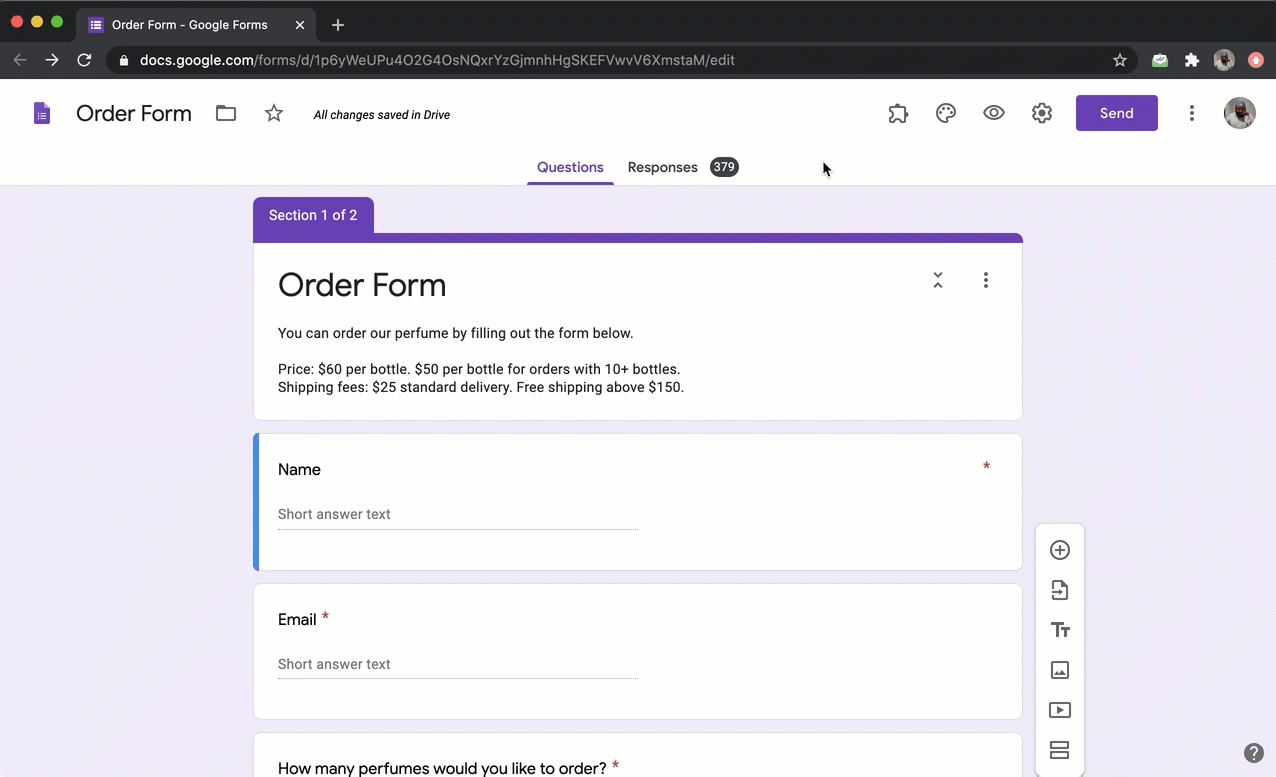#### Simple calculation

We have to add the order amount and shipping fees to calculate the total amount. It's a simple addition.
Order amount + Shipping fees

In the Formfacade customize interface, click on the gear icon next to the Total amount field. Field settings screen will be displayed. Select the Answer tab and write the above formula in the Calculate option.

To use a form field in the calculation, type @ and you will see the list of all the fields in the form. Select the required form field to add the field. You will see the display name of the field, but internally it uses the entry id for the calculations.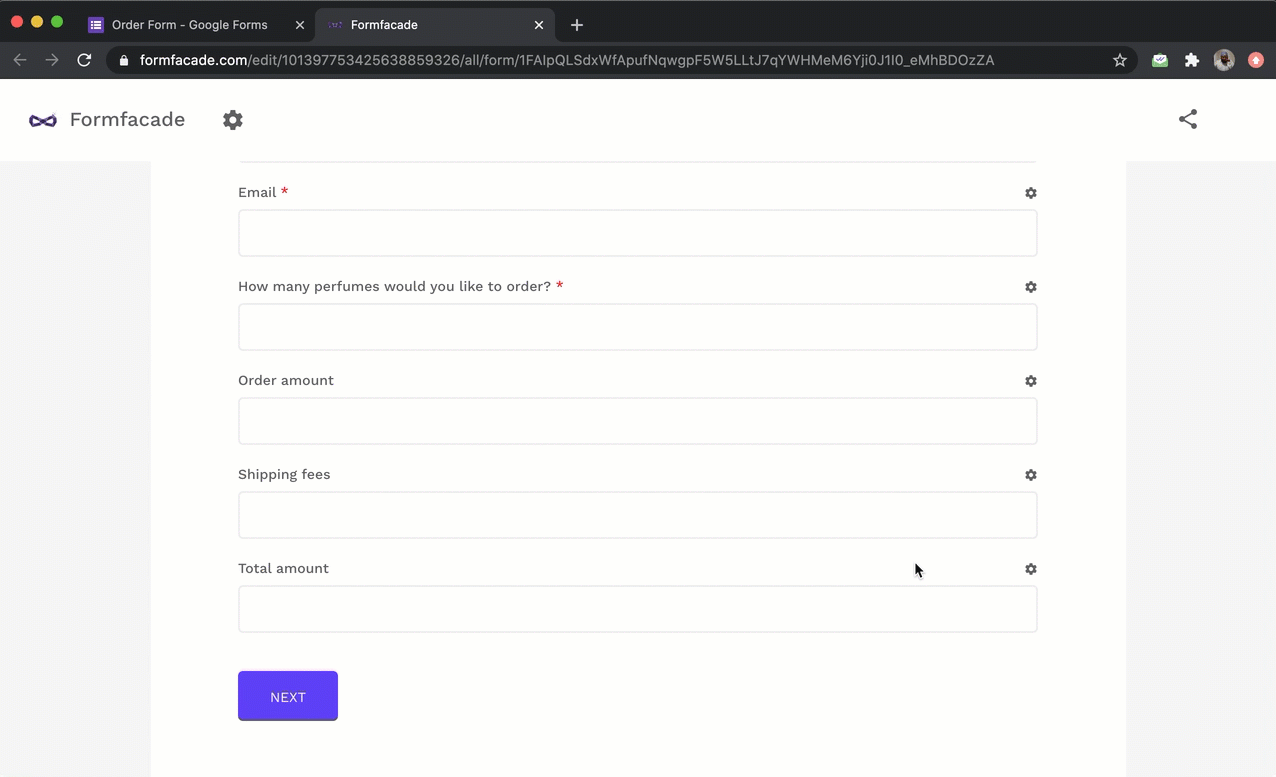#### Conditional logic

The standard shipping fee is \$25, but this fees is waived off for orders above \$150. To implement this calculation, we have to check if the order amount is greater than 150.

You can use the IFS function to write conditional logic. IFS function evaluates multiple conditions and returns a value that corresponds to the first true condition. The syntax for the IFS function is:

IFS(condition1, value1, [condition2, value2, …])
where

• condition1 - The first condition to be evaluated.
• value1 - The returned value if condition1 is TRUE.
• condition2, value2, … - Additional conditions and values if the first one is evaluated to be false.

The formula to calculate the shipping fees is:
IFS(Order Amount > 150, 0, 25)

In the Formfacade customize interface, click on the gear icon next to the Shipping fees field. Field settings screen will be displayed. Select the Answer tab and write the above IFS formula in the Calculate option.

Note: To use a form field in the calculation, type @ and you will see the list of all the fields in the form. Select the required form field to add the field. You will see the display name of the field, but internally it uses the entry id for the calculations.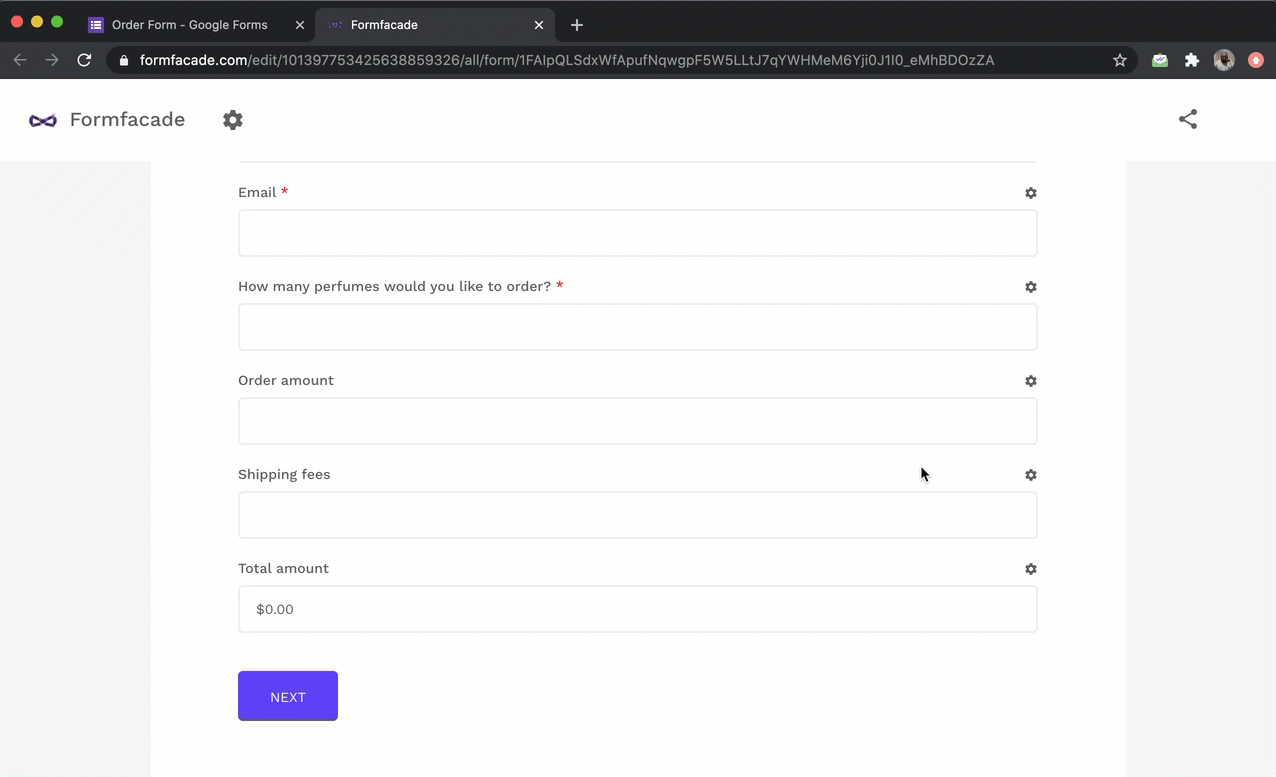#### Conditional logic + Calculations

Order amount = Quantity * Price

Quantity is entered by the user. Based on this quantity entered, we have to calculate the price. The standard price is \$60, but if a user orders 10 or more bottles of the perfume, the price per bottle is \$50. We can use the IFS function to determine price and then multiply it with quantity to calculate the order amount.

IFS(Quantity >=10 , 50, 60) * Quantity

In the Formfacade customize interface, click on the gear icon next to the Order amount field. Field settings screen will be displayed. Select the Answer tab and write the above formula in the Calculate option.

Note: To use a form field in the calculation, type @ and you will see the list of all the fields in the form. Select the required form field to add the field. You will see the display name of the field, but internally it uses the entry id for the calculations.